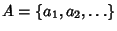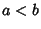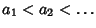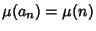## Möbius Problem

Letbe a free Abelian Semigroup, whereis the unit element, and letbe the Möbius Function. Then do the following properties,

1.Impliesfor, wherehas the linear order,

2.for all,
imply thatfor all? The problem is known to be true forfor all.

Flath, A. and Zulauf, A. Does the Möbius Function Determine Multiplicative Arithmetic?'' Amer. Math. Monthly 102, 354-256, 1995.